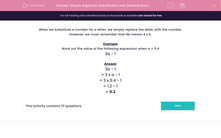# Simple Algebraic Substitution with Decimal Numbers

In this worksheet, students substitute decimal numbers for letters and work out the value of simple expressions with one variable.Key stage:  KS 2

Curriculum topic:   Maths and Numerical Reasoning

Curriculum subtopic:   Equations and Algebra

Difficulty level:#### Worksheet Overview

When we substitute a number for a letter, we simply replace the letter with the number.

However, we must remember that 4b means 4 x b.

Example

Work out the value of the following expression when a = 0.4

3a - 1

3a - 1

= 3 x a - 1

= 3 x 0.4 - 1

= 1.2 - 1

= 0.2

### What is EdPlace?

We're your National Curriculum aligned online education content provider helping each child succeed in English, maths and science from year 1 to GCSE. With an EdPlace account you’ll be able to track and measure progress, helping each child achieve their best. We build confidence and attainment by personalising each child’s learning at a level that suits them.

Get started